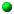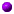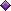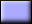# Selective Execution### Select the topics you wish to review:Selective ExecutionLOGICAL Type and VariablesLOGICAL Input and OutputRelational OperatorsLOGICAL Operators and ExpressionsIF-THEN-ELSE-END IF StatementForm 1: IF-THEN-ELSE-END IFForm 2: IF-THEN-END IFForm 3: Logical IFProgramming Example 1: Quadratic Equation SolverProgramming Example 2: Final Mark ComputationProgramming Example 3: Heron's Formula for Computing Triangle AreaNested IF-THEN-ELSE-END IFIF-THEN-ELSE IF-END IFProgramming Examples:Quadratic Equation Solver: AgainQuadratic Equation Solver: RevisitedSort Three NumbersSELECT CASE StatementProgramming Example 1: Computing Letter GradeProgramming Example 2: Character TestingDownload my course overheads